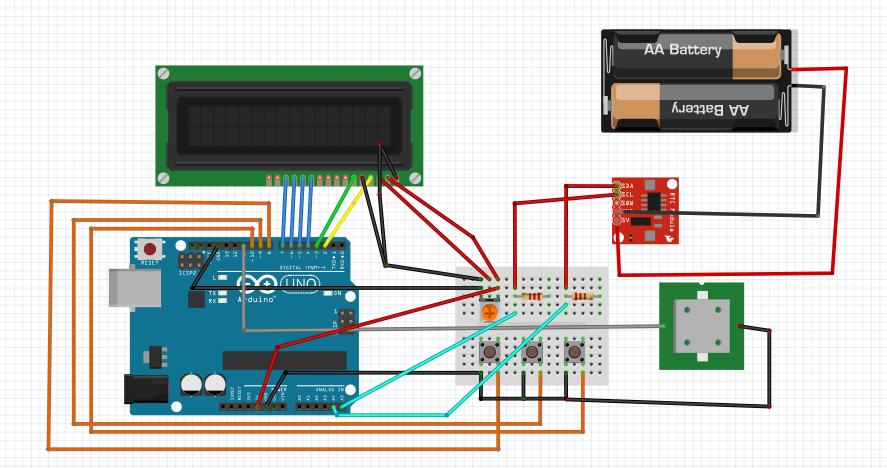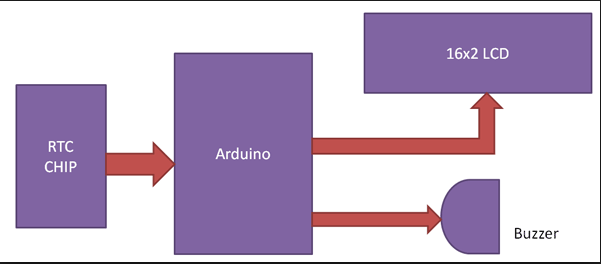Arduino Alarm Clock – Zero Ohm Electronics

# Arduino Alarm Clock

Posted by Momen Odeh on

Arduino Alarm Clock

In this tutorial, I will try to explain how to implement a Real Time Clock (RTC) with alarm which is a battery powered clock that measures power even when there is no external power or the microcontroller is reprogrammed.

The battery which is connected to the RTC is a separate one and is not related or connected to the main power supply. An RTC displays clock and calendar with all timekeeping functions. Below are the hardware components required for this project.

Hardware Components:

• Arduino UNO
• 3 Push Buttons
• Buzzer
• DS 1307 RTC Module
• 16x2 LCD Display
• 2 10K resistors
• 10K potentiometer

Now onto the hardware description!

Hardware Description

I will give you a brief introduction about DS 1307 first and then a summary of the circuit design.

DS 1307 is basically the frequency used RTC IC for clock and calendar. The clock function provides seconds, minutes and hours while the calendar function provides day, date, month and year values. The clock can either operate in 12 hour with AM/PM indication or 24-hour format. A 3V backup battery must be connected to the RTC so that the IC can automatically switch to backup supply in case of power failure.

Now let’s talk about the circuit. The communication between the microcontroller (UNO) and DS 1307 is serial I2 C bidirectional bus. There are two pins on Arduino for I2 C communication. Analog pins 4 and 5 will act as SDA (Serial Data) and SCL (Serial Clock). These are connected to the SDA and SCL pins of DS 1307. Both of these pins are pulled high using 10K resistors. An LCD is used to display the clock. 6 pins of LCD must be connected to UNO, RS, E, D5, D6, D7 (Pins 4,6,11,12,13 and 14) of LCD are connected to pins 2,3,4,5,6 and 7 of UNO. Three buttons are used to set the alarm. These pins are connected to pins 8,9 and 10 of UNO. A buzzer is connected to pin 11 of UNO and acts as an alarm. Below is the hardware overview of the project.NOTE: I have assumed that you are aware of the communication protocol we are using here and the DS 1307 module requires 5V battery but I have used 3V battery here for demonstration purposes.

We can now focus in the software design!

Software Description

Pins 8,9 and 10 as you know represent the pushbuttons. Pin 8 represents the NEXT pushbutton, pin 9 the INCREMENT pushbutton and pin 10 represents the SET pushbutton. The working of the code is explained in the below points.

1. The code will be uploaded to UNO and the LCD will display the current date and time.
2. In order for the alarm to be set, the SET pushbutton must be pressed which will make it go alarm mode. It will ask for hours with current time being displayed, the INCREMENT button be pressed to change the hours.
3. The clock is 24 hour format so the hours will be incremented between 0 and 23. Once the hours of the alarm is set we will go to the minutes by pressing the NEXT button.
4. The INCREMENT button is pressed again to change the minutes. SET button is pressed again and the alarm will be set.
5. The values entered as alarm are stored in EEPROM of the UNO. These values are compared continuously with the present time.
6. When stored values matches the current value, the buzzer will go off. In order to stop the alarm, the NEXT button is pressed.

Below is the code for the whole project.

Libraries

#include <Wire.h>
#include<EEPROM.h>
#include <RTClib.h>
#include <LiquidCrystal.h>
LiquidCrystal lcd(2, 3, 4, 5, 6, 7);
RTC_DS1307 RTC;
int tmp,Inc,hor,mIn,add=11;
int set=8;
int cge=9;
int mod=10;
int off=0;
#define buz 11
int Hor,Min,Sec;
Time Adjustment
void time()
{
int tmp=1,mins=0,hors=0,secs=0;
while(tmp==1)
{
off=0;
if(digitalRead(cge)==0)
{
Hor++;
if(Hor==24)
{
Hor=0;
}
}
lcd.clear();
lcd.setCursor(0,0);
lcd.print("Set Alarm Time ");
lcd.setCursor(0,1);
if(Hor<=9)
lcd.print("0");
lcd.print(Hor);
lcd.print(":");
lcd.print(Min);
lcd.print(":");
lcd.print(Sec);
delay(200);
lcd.setCursor(0,1);
lcd.print("  ");
lcd.print(":");
lcd.print(Min);
lcd.print(":");
lcd.print(Sec);
delay(200);
if(digitalRead(set)==0)
{
hor=Hor;
EEPROM.write(add++,hor);
tmp=2;
while(digitalRead(set)==0);
}
}

while(tmp==2)
{
if(digitalRead(cge)==0)
{
Min++;
if(Min==60)
{Min=0;}
}
lcd.setCursor(0,1);
lcd.print(Hor);
lcd.print(":");
if(Min<=9)
lcd.print("0");
lcd.print(Min);
lcd.print(":");
lcd.print(Sec);
lcd.print("  ");
delay(200);
lcd.setCursor(0,1);
lcd.print(Hor);
lcd.print(":");
lcd.print("  ");
lcd.print(":");
lcd.print(Sec);
lcd.print("  ");
delay(200);
if(digitalRead(set)==0)
{
mIn=Min;
EEPROM.write(add++, mIn);
tmp=0;
while(digitalRead(set)==0);
}
}
off=1;
delay(10);
}
Sounding the buzzer
void Buz()
{
if(digitalRead(set)==0)
off=0;
if(off==1)
{
digitalWrite(buz,HIGH);
delay(500);

digitalWrite(buz, LOW);
delay(500);
}
}
Comparison between current and stored time
void TimeCheck()
{
int tem;
for(int i=11;i<17;i++)
{
tem[i]=EEPROM.read(i);
}
if(Hor == tem && Min == tem && off==1)
{
add=11;
Buz();
Buz();
lcd.clear();
lcd.print("alarm...........");
lcd.setCursor(0,1);
lcd.print("...........alarm");
Buz();
Buz();

## Leave a comment

Please note, comments must be approved before they are published.

Liquid error: Could not find asset snippets/bold-currency-converter.liquid